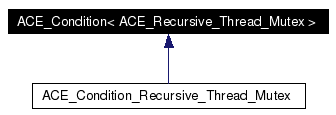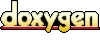# ACE_Condition< ACE_Recursive_Thread_Mutex > Class Template Reference

ACE_Condition template specialization written using ACE_Recursive_Thread_Mutex. This allows threads to block until shared data changes state using recursive mutexes. More...

`#include <Condition_Recursive_Thread_Mutex.h>`

Inheritance diagram for ACE_Condition< ACE_Recursive_Thread_Mutex >:[legend]
Collaboration diagram for ACE_Condition< ACE_Recursive_Thread_Mutex >:[legend]
List of all members.

## Public Member Functions

Initialize the condition variable with a recursive mutex.

~ACE_Condition (void)
Implicitly destroy the condition variable.

int remove (void)
int wait (const ACE_Time_Value *abstime=0)
int wait (ACE_Recursive_Thread_Mutex &mutex, const ACE_Time_Value *abstime=0)
int signal (void)

Returns a reference to the underlying mutex;.

void dump (void) const
Dump the state of an object.

## Private Member Functions

void operator= (const ACE_Condition< ACE_Recursive_Thread_Mutex > &)
ACE_Condition (const ACE_Condition< ACE_Recursive_Thread_Mutex > &)

## Private Attributes

ACE_cond_t cond_
A normal (i.e., non-recursive) condition variable.

Reference to the recursive mutex.

## Detailed Description

### template<> class ACE_Condition< ACE_Recursive_Thread_Mutex >

ACE_Condition template specialization written using ACE_Recursive_Thread_Mutex. This allows threads to block until shared data changes state using recursive mutexes.

Definition at line 42 of file Condition_Recursive_Thread_Mutex.h.

## Constructor & Destructor Documentation

 ACE_Condition< ACE_Recursive_Thread_Mutex >::~ACE_Condition ( void )
 Implicitly destroy the condition variable. Definition at line 44 of file Condition_Recursive_Thread_Mutex.cpp. References ACE_Condition< MUTEX >::remove(). ```00045 { 00046 this->remove (); 00047 } ```

## Member Function Documentation

 ACE_Condition< ACE_Recursive_Thread_Mutex >::ACE_Condition ( const ACE_Condition< ACE_Recursive_Thread_Mutex > & ) ` [private]`

 Initialize the condition variable with a recursive mutex. Definition at line 49 of file Condition_Recursive_Thread_Mutex.cpp. References ACE_OS::cond_init(). ```00050 : mutex_ (m) 00051 { 00052 ACE_OS::cond_init (&this->cond_); 00053 } ```

 Signal *all* waiting threads. Definition at line 110 of file Condition_Recursive_Thread_Mutex.cpp. References ACE_OS::cond_broadcast(). Referenced by ACE_Future_Rep< T >::set(). ```00111 { 00112 return ACE_OS::cond_broadcast (&this->cond_); 00113 } ```

 void ACE_Condition< ACE_Recursive_Thread_Mutex >::dump ( void ) const
 Dump the state of an object. Definition at line 30 of file Condition_Recursive_Thread_Mutex.cpp. References ACE_BEGIN_DUMP, ACE_DEBUG, ACE_END_DUMP, ACE_LIB_TEXT, and LM_DEBUG. Referenced by ACE_Future_Rep< T >::dump(). ```00031 { 00032 #if defined (ACE_HAS_DUMP) 00033 // ACE_TRACE ("ACE_Condition::dump"); 00034 00035 ACE_DEBUG ((LM_DEBUG, ACE_BEGIN_DUMP, this)); 00036 // No dump method for ACE_cond_t even in emulated mode. 00037 // cond_.dump (); 00038 this->mutex_.dump (); 00039 ACE_DEBUG ((LM_DEBUG, ACE_LIB_TEXT ("\n"))); 00040 ACE_DEBUG ((LM_DEBUG, ACE_END_DUMP)); 00041 #endif /* ACE_HAS_DUMP */ 00042 } ```

 Returns a reference to the underlying mutex;. Definition at line 116 of file Condition_Recursive_Thread_Mutex.cpp. ```00117 { 00118 return this->mutex_; 00119 } ```

 void ACE_Condition< ACE_Recursive_Thread_Mutex >::operator= ( const ACE_Condition< ACE_Recursive_Thread_Mutex > & ) ` [private]`

 ACE_BEGIN_VERSIONED_NAMESPACE_DECL int ACE_Condition< ACE_Recursive_Thread_Mutex >::remove ( void )
 Explicitly destroy the condition variable. Note that only one thread should call this method since it doesn't protect against race conditions. Definition at line 24 of file Condition_Recursive_Thread_Mutex.cpp. References ACE_OS::cond_destroy(). ```00025 { 00026 return ACE_OS::cond_destroy (&this->cond_); 00027 } ```

 int ACE_Condition< ACE_Recursive_Thread_Mutex >::signal ( void )
 Signal one waiting thread. Definition at line 104 of file Condition_Recursive_Thread_Mutex.cpp. References ACE_OS::cond_signal(). ```00105 { 00106 return ACE_OS::cond_signal (&this->cond_); 00107 } ```

 int ACE_Condition< ACE_Recursive_Thread_Mutex >::wait ( ACE_Recursive_Thread_Mutex & mutex, const ACE_Time_Value * abstime = 0 )
 Block on condition or until absolute time-of-day has passed. If abstime == 0 use "blocking" wait() semantics on the recursive mutex passed as a parameter (this is useful if you need to store the in shared memory). Else, if != 0 and the call times out before the condition is signaled returns -1 and sets errno to ETIME. Definition at line 62 of file Condition_Recursive_Thread_Mutex.cpp. ```00064 { 00065 ACE_recursive_mutex_state mutex_state_holder; 00066 ACE_recursive_thread_mutex_t &recursive_mutex = mutex.mutex (); 00067 00068 if (ACE_OS::recursive_mutex_cond_unlock (&recursive_mutex, 00069 mutex_state_holder) == -1) 00070 return -1; 00071 00072 // We wait on the condition, specifying the nesting mutex. For platforms 00073 // with ACE_HAS_RECURSIVE_MUTEXES, this is the recursive mutex itself, 00074 // and is the same as recursive_mutex, above. The caller should have been 00075 // holding the lock on entry to this method, and it is still held. 00076 // For other platforms, this is the nesting mutex that guards the 00077 // ACE_recursive_mutex_t internals, and recursive_mutex_cond_unlock() 00078 // returned with the lock held, but waiters primed and waiting to be 00079 // released. At cond_wait below, the mutex will be released. 00080 // On return, it will be reacquired. 00081 const int result = abstime == 0 00082 ? ACE_OS::cond_wait (&this->cond_, 00083 &mutex.get_nesting_mutex ()) 00084 : ACE_OS::cond_timedwait (&this->cond_, 00085 &mutex.get_nesting_mutex (), 00086 const_cast (abstime)); 00087 // We are holding the mutex, whether the wait succeeded or failed. 00088 // Stash errno (in case it failed) and then we need to reset the 00089 // recursive mutex state to what it was on entry to this method. 00090 // Resetting it may require a wait for another thread to release 00091 // the ACE_recursive_thread_mutex_t if this is a platform without 00092 // ACE_HAS_RECURSIVE_MUTEXES, and recursive_mutex_cond_relock() takes 00093 // care of that. 00094 { 00095 ACE_Errno_Guard error (errno); 00096 ACE_OS::recursive_mutex_cond_relock (&recursive_mutex, 00097 mutex_state_holder); 00098 } 00099 00100 return result; 00101 } ```

 int ACE_Condition< ACE_Recursive_Thread_Mutex >::wait ( const ACE_Time_Value * abstime = 0 )
 Block on condition, or until absolute time-of-day has passed. If abstime == 0 use "blocking" semantics. Else, if != 0 and the call times out before the condition is signaled returns -1 and sets errno to ETIME. Definition at line 56 of file Condition_Recursive_Thread_Mutex.cpp. References ACE_Condition< MUTEX >::wait(). Referenced by ACE_Future_Rep< T >::get(), and ACE_Future_Rep< T >::operator T(). ```00057 { 00058 return this->wait (this->mutex_, abstime); 00059 } ```

## Member Data Documentation

 ACE_cond_t ACE_Condition< ACE_Recursive_Thread_Mutex >::cond_` [private]`
 A normal (i.e., non-recursive) condition variable. Definition at line 98 of file Condition_Recursive_Thread_Mutex.h.

 ACE_Recursive_Thread_Mutex& ACE_Condition< ACE_Recursive_Thread_Mutex >::mutex_` [private]`
 Reference to the recursive mutex. Definition at line 101 of file Condition_Recursive_Thread_Mutex.h.

The documentation for this class was generated from the following files:
Generated on Thu Nov 9 11:20:46 2006 for ACE by1.3.6